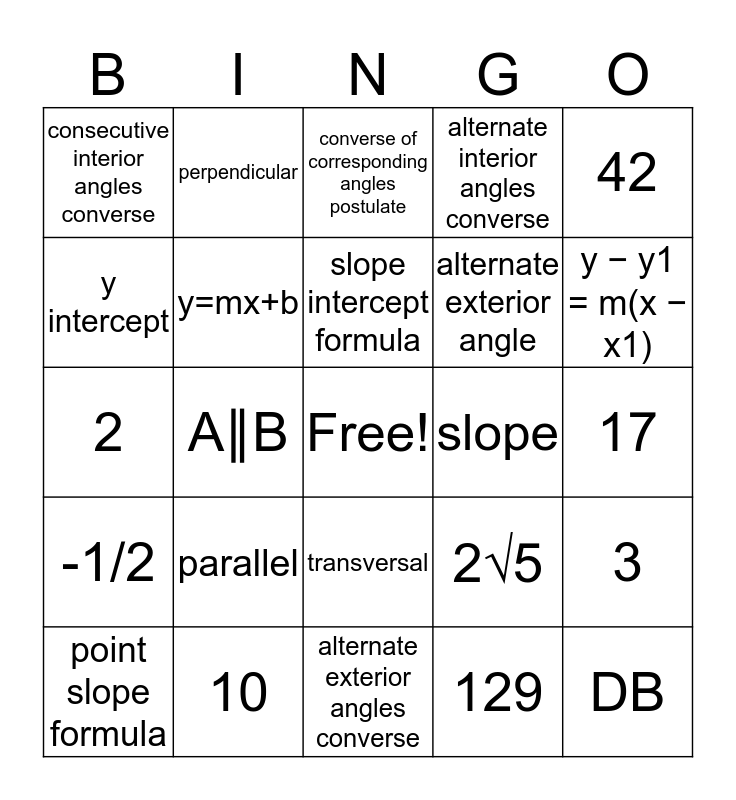# Geometry Chapter 3 BingoThis bingo card has a free space and 24 words: parallel, 10, slope intercept formula, point slope formula, 42, consecutive interior angles converse, y intercept, perpendicular, slope, transversal, alternate exterior angle, 2, 2√5, 3, y=mx+b, 129, 17, converse of corresponding angles postulate, y − y1 = m(x − x1), A∥B, DB, -1/2, alternate interior angles converse and alternate exterior angles converse.

## Play Online